# AP Calculus AB Practice Test 22

### Test Information10 questions20 minutes

Calculator Disallowed

1.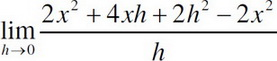2. Find the point on the curve x2 + y2 = 9 that is a minimum distance from the point (1, 2).

3. Find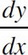If y = log3(2x3 + 4x2).

4. What curve is represented by x = 2t3 and y = 4t9?

5. Find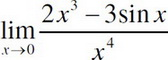.

6.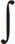18x2sec2(3x3)dx=

7. What is the equation of the line normal to the curve y = x3 + 2x2 - 5x + 7 at x = 1 ?

8.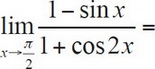9. If cos2x + sin2y = y, then.

10. If f(x) = e3x, then f′′(ln3) =